Next: The authentication property Up: CSP, PVS and a Previous: A key theorem

# Translating to PVS notation

In [DS97a], an embedding of CSP in PVS is presented, precisely for mechanising the proofs necessary with this approach. CSP traces are represented as lists, a pre-defined notion in PVS. Processes are described as sets of traces. The CSP operators are then defined as trace combinators. For example, the choice operator `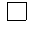' returns the union of its two arguments.

Since a process P satisfies a predicate E iff all its traces satisfy E , a satisfaction operator ``|>`' can be defined, so that P `|>` E provided P is a subset of E .

The Dolev-Yao framework has already been translated into PVS [DS97a,BS97], so all that was required was to define the message space and the protocol agents.

The message space was defined as

```message : DATATYPE WITH SUBTYPES nonkey, key
BEGIN
text     (x_text     : Text)              : text?  : nonkey
nonce    (x_nonce    : Nonce)             : nonce? : nonkey
user     (x_user     : Identity)          : user?  : nonkey
conc     (x_conc, y_conc : message)       : conc?  : nonkey
session  (x_session  : SessionKey)        : session?  : key
longterm (x_longterm : LongTerm)          : longterm? : key
code     (x_code : key, y_code : message) : code?  : nonkey
hash     (x_hash : key, y_hash : message) : hash?  : nonkey
END message
```
The message authentication code is defined by
``` mac(k, m) : message =  conc(hash(k, m), m)
```

The enemy may deduce certain information from the messages it sees. This deductive ability, given by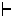in the CSP model, is transcribed into PVS by the `Gen` relation:

```  Gen(S)(m) : INDUCTIVE bool =
0)       S(m)
1) OR (EXISTS m1, m2: Gen(S)(m1) AND Gen(S)(m2) AND m = conc(m1, m2))
2) OR (EXISTS m1: Gen(S)(conc(m1, m)))
3) OR (EXISTS m2: Gen(S)(conc(m, m2)))
4) OR (EXISTS m1, k: Gen(S)(m1) AND Gen(S)(k) AND m = crypto(k, m1))
5) OR (EXISTS k: Gen(S)(k) AND Gen(S)(crypto(k, m)))
6) OR (EXISTS m1, k: Gen(S)(m1) AND Gen(S)(k) AND m = hash(k, m1));
```

Any message already known to the enemy is considered to be part of the generated set (line 0). The enemy may concatenate messages, or split concatenated messages (lines 1-3). If it is in possession of a key and an arbitrary message, it may encrypt the message with the key. (line 4). Since all keys are symmetric, if it owns a key and a message encrypted with that key, it may decrypt the message (line 5). Finally, if it owns a key and an arbitrary message, it may form the hash of the message with respect to the key (line 6). The transitivity requirement is implicit, because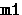,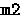and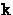are quantified over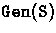.

With these in place, it now remains to prove that each of the protocol participants maintain rank. This means that if the CSP description of an individual participant is restricted, so it cannot transmit any messages from the set R , then it is unable to transmit the message T . The contrapositive of this says that if the message T is observed on the medium, it must have been preceded by an event from the set R .

We need to define an operator `crypto`, which will encrypt and decrypt messages.

```crypto(k, m) : message =
CASES m OF
code(x, y) :
CASES k OF
longterm(i): IF x = longterm(i)  THEN y ELSE code(k, m) ENDIF,
session(i): IF x = session(i) THEN y ELSE code(k, m) ENDIF
ENDCASES
ELSE code(k, m)
ENDCASES
```

It applies the function `code`, returning the original message if the key has been applied twice to the same message.

In the following definitions, `lt` is an abbreviation for the function which returns the longterm key of a user `i`, and `sk(i,j)` returns the session key for `i` and `j`.

The first user is defined as:

``` userA : process[event] =
Choice! skey :
( trans(a, b, mac(lt(a), conc4(Ia, Ib, Na, null))) >>
(   rec(a, b, crypto(lt(a), conc3(skey, Ib, Na))) >>
Stop[event]   )
```

The `Choice! skey` means that in the second part of the definition, agent A is prepared to accept an arbitrary session key, provided that it forms part of a certificate encrypted with its longterm key, and contains his original nonce.

The definition of `userB` is similar, except that it first waits for a message from `userA`, then uses that message instead of the placeholder. It also expects two messages, each containing a single key certificate, and does not pass anything on to the lower members of the chain. These changes were necessitated by the definition of the server.

``` userB : process[event] =
Choice! ltk, Nx, m, skup, skdown, i, k :
(rec(b, i, mac(ltk, conc4(user(i), Ib, Nx, m))) >>
( trans(b, k, mac(lt(b), conc4(Ib, user(k), Nb,
mac(ltk, conc4(user(i), Ib, Nx, m))))) >>
( rec(b, k, crypto(lt(b), conc3(skup, user(k), Nb))) >>
( rec(b, k, crypto(lt(b), conc3(skdown, user(i), Nb))) >>
Stop[event] ))))
```
The definition of the server differs in some ways from its CSP definition. In the CSP definition, the server receives one message, and sends out one message. However, a definition of this form would require the definition of `response ' to be incorporated into the definition of the server.. This would require significant extra complexity in the PVS coding. It proved easier to make use of the assumptions of the Dolev-Yao model.

Since the medium is entirely in the control of the enemy, who may reorder, redirect or kill messages arbitrarily, we do not need to define the server to recurse on a single message to produce all the certificates. The inner layers of the message have already been circulating in the medium, and we may therefore rely on the medium to pass these on to the server as appropriate.

This is not as radical an assumption as it may seem, and it introduces no further attacks on the protocol. The medium may already destroy any run of the protocol by refusing to pass on messages. But what we are interested in are safety properties: if a protocol run completes successfully, then we want to be sure that the session keys are uncompromised. Provided it is possible for a single run to complete successfully, we are not interested in any incomplete runs.

In this description, the server receives a message, which either has at least two levels of message authentication codes, or contains the placeholder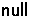. The two parts of the definition result from the pattern matching that occurs on the first message. It the message contains at least two levels of message authentication codes, then the server prepares and sends the appropriate two key certificates. These are addressed direct to the intended recipient: since our enemy may arbitrarily redirect messages, nothing is gained by insisting that they pass through all members of the chain.

If the incoming message contains the placeholder, then only one certificate is necessary.

```Fs(X) : process[event] =
(Choice! m2, Nix, Njx, i, j, k, l :
(rec(s,l,mac(lt(j), conc4(user(j), user(k), Njx,
mac(lt(i), conc4(user(i), user(j), Nix, m2))))) >>
( trans(s, j, crypto(lt(j), conc3(sk(j,k), user(k), Njx))) >>
(  trans(s, j, crypto(lt(j), conc3(sk(j,i), user(i), Njx))) >>
X))))
\/
(Choice! Nix, i, j, l :
( rec(s, l, mac(lt(i), conc4(user(i), user(j), Nix, null))) >>
(  trans(s, l, mac(lt(i), conc4(user(i), user(j), Nix, null))) >>
(   Stop[event]))))

server : process[event] = mu(Fs)
```Next: The authentication property Up: CSP, PVS and a Previous: A key theorem